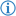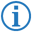The less than or equal operator is a type of comparison operator which returns the boolean value `<literal:true>` if the first number object's value is smaller than the second's or equal to it, else returns `<literal:false>`.

Its token is named LESS_THAN_OR_EQUAL.

## FDS`<value:number>``<whitespace>``<comparator>``[no/not/n't] [greater/more] than``<whitespace>``<value:number>`

### Example

`If`` ``Spike's age`` ``is no more than`` ``2`` ``then``:` `...`

This executes the next part of code only if the variable `Spike's age` is smaller than `8` or equal to it.

This is equivalent to the following Java code:

```if(Spike_s_age<=8) { ...
```